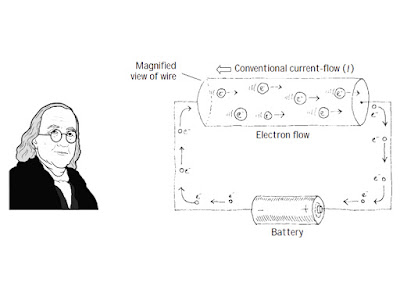Basic Electronics All About Electrical Current.Electrical Current

Electrical Current:

Electrical charge flows freely in certain materials, called conductors, but not in others, called insulators. Metals and a few other elements and compounds are good conductors, while air,

glass, plastics, and rubber are insulators. In addition, there is a third category of materials called semiconductors; sometimes they seem to be good con-ductors but much less so other times. Silicon and Germanium are two such semiconductors.Figure 1

Figure 1 shows two connected, oppositely charged bodies. The force betweenthem has the potential for work; therefore, there is voltage. If the two bodies are connected by a conductor, as in the illustration, the positive charge moves along the wire to the other sphere. On the other end, the negative charge flows out on the wire towards the positive side. In this case, positive and negative charges combine to neutralize each other until there are no charge differences between any points in the system.

The flow of an electrical charge is called a current. Current is measured in amperes (a), also called amps, after Andre Ampere, a French mathematician and physicist. An ampere is defined as a flow of one Coulomb of charge in one second.

Electrical current is directional; therefore, a positive current is the flow current from a positive point A to a negative point B. However, most current results from the flow of negative-to-positive charges.

Current (symbolized with an I) represents the amount of electrical charge ∆Q (or dQ) crossing a cross-sectional area per unit time, which is given by:

I = ΔQ/Δt = dQ/dt

The unit of current is called the ampere (abbreviated amp or A) and is equal to one coulomb per second:

1A = 1C/S

Electric currents typically are carried by electrons. Each electron carries a charge of −e, which equals:

-e = 1.6×10^(-19)C

Benjamin Franklin’s Positive Charges

Now, there is a tricky, if not crude, subtlety with regard to the direction of current flow that can cause headaches and confusion later on if you do not realize a historical convention initiated by Benjamin Franklin (often considered the father of electronics). Anytime someone says “current I flows from point A to point B,” you would undoubtedly assume, from what I just told you about current, that electrons would flow from point A to point B, since they are the things moving. It seems obvious. Unfortunately, the conventional use of the term current, along with the symbol I used in the equations, assumes that positive charges are flowing from point A to B! This means that the electron flow is, in fact, pointing in the opposite direction as the current flow. What’s going on? Why do we do this?

The answer is convention, or more specifically, Benjamin Franklin’s convention of assigning positive charge signs to the mysterious things (at that time) that were moving and doing work. Sometime later a physicist by the name of Joseph Thomson performed an experiment that isolated the mysterious moving charges. However, to measure and record his experiments, as well as to do his calculations, Thomson had to stick with using the only laws available to him—those formulated using Franklin’s positive currents. However, these moving charges that Thomson found (which he called electrons) were moving in the opposite direction of the conventional current I used in the equations, or moving against convention.Figure 2

What does this mean to us, to those of us not so interested in the detailed physics and such? Well, not too much. I mean that we could pretend that there were positive charges moving in the wires, and electrical devices and things would work out fine. In fact, all the formulas used in electronics, such as Ohm’s law (V = IR), “pretend” that the current I is made up of positive charge carriers. We will always be stuck with this convention. In a nutshell, whenever you see the term current or the symbol I, pretend that positive charges are moving. However, when you see the term electron flow, make sure you realize that the conventional current flow I is moving in the opposite direction.

Measuring current:Figure 3

Figure 3 Measuring current. (a) Schematic diagram of series circuit consisting of power supply, 10 k potentiometer, and multimeter. (Note that the center tap of the potentiometer is left unconnected in this exercise – accidentally connecting it to power or ground could lead to excessive current flow and burn out the pot.) (b) A drawing of the same circuit showing how the DMM leads should be configured to measure current. Note that the meter is connected in series with the resistor.